## NCERT Solutions for Class 10 Science Chapter 1 Chemical Reactions and Equations

In this chapter students will learn about writing chemical equations, balancing chemical equations, different types of chemical equations, decomposition reaction, displacement reaction, double displacement reaction, oxidation and reaction, corrosion, rancidity.

These Solutions are part of NCERT Solutions for Class 10 Science. Here we have given NCERT Solutions for Class 10 Science Chapter 1 Chemical Reactions and Equations. LearnInsta.com provides you the Free PDF download of NCERT Solutions for Class 10 Science (Chemistry) Chapter 1 – Chemical Reactions and Equations solved by Expert Teachers as per NCERT (CBSE) Book guidelines. All Chapter 1 – Chemical Reactions and Equations Exercise Questions with Solutions to help you to revise complete Syllabus and Score More marks.

### NCERT Solutions for Class 10 Science Chapter 1 In Text Book Questions

Question 1.
Why should magnesium ribbon be cleaned before burning in air ?
Magnesium ribbon has generally a coating of basic magnesium carbonate on its surface. It is a mixture of magnesium hydroxide and magnesium carbonate and is slowly deposited on the surface of the metal by the action of moist air. The coating or layer prevents the metal from burning when flame is brought in contact with the metal. The surface should be properly cleaned preferably with a sand paper before burning the ribbon in air in order to remove the layer of magnesium oxide.

More Resources

Question 2.
Write the balanced equations for the following chemical reactions :
(i) Hydrogen + Chlorine ———> Hydrogen chloride
(ii) Sodium + Water ———> Sodium hydroxide + Hydrogen
(iii) Barium chloride + Aluminium sulphate ———> Barium sulphate + Aluminium chloride.
The balanced equations are written for the symbol equations and not for word equations.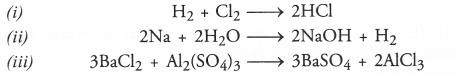Question 3.
Write the balanced equations with state symbols for the following reactions :
(i) Solutions of barium chloride and sodium sulphate in water react to give insoluble barium sulphate and solution of sodium chloride.
(ii) Sodium hydroxide solution (in water) reacts with hydrochloric acid solution (in water) to produce sodium chloride (in water) and water.
The symbol equations in balanced form for the reactions are :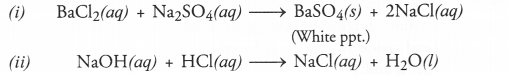Question 4.
A solution of the substance ‘X’ is used for white washing.
(i) Name the substance ‘X’ and write its formula.
(ii) Write the reaction of the substance ‘X’ with water.
(i) The substance lX’ is calcium oxide (also called quick lime). Its formula is CaOQuestion 5.
With the help of an experiment show that in the electrolysis of acidulated water, the volume of one gas is twice the volume of the other gas. Name the gas.
The gas is hydrogen and its volume is twice that of oxygenQuestion 6.
When you mix solutions of lead (II) nitrate and potassium iodide,
(i) What is the colour of the precipitate formed ? Name the compound involved.
(ii) Write a balanced chemical equation for the reaction
(iii) Is this a double displacement reaction ?
(i) The precipitate is yellow in colour. The compound is lead (II) iodide with chemical formula Pbl2.
(ii) pb(NO3)(aq) + 2KI (aq) ———-> Pbl(s) + 2KNO(aq)
(iii) Yes, it is a double displacement reaction.

Question 7.
Why does the colour of copper sulphate change when an iron nail is dipped in it ?
Iron nail acquires a brown coating of copper as a result of the displacement reaction in which iron has displaced copper from copper sulphate solution. The solution becomes light green.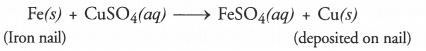Question 8.
Give one example of the double displacement reaction.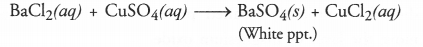Question 9.
Identify the substances that are oxidised and the substances that are reduced in the following reactions :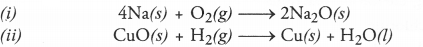(i) In this reaction, sodium (Na) is oxidised to sodium oxide (Na2O). This means that oxygen (O2) has been reduced.
(ii) In this reaction, hydrogen (H2) is oxidised to water (H2O) while copper (II) oxide (CuO) is reduced to copper (Cu).

Question 10.
Magnesium ribbon burns with a dazzling flame in air (or oxygen) and changes to white substance magnesium oxide. Is magnesium being oxidised or reduced in this reaction ?
The balanced chemical equation for the reaction is :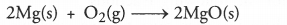Magnesium (Mg) is oxidised to magnesium oxide (MgO) in this reaction.

### NCERT Solutions for Class 10 Science Chapter 1 NCERT End Exercises

Question 1.
WTiich of the following statements about the reaction given below are incorrect ?(b) Carbon dioxide is getting oxidised
(c) Carbon is getting oxidised
(d) Lead oxide is getting reduced
(i) a and b
(ii) a and c
(iii) a, b and c
(iv) all are incorrect
(i) a and b are both incorrect
Pb is getting oxidised to PbO in backward reaction.
CO2 is getting reduced to C in backward reaction.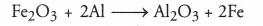Question 2.
The above reaction is an example of :
(a) combination reaction
(b) double displacement reaction
(c) decomposition reaction
(d) displacement reaction
(d). It is an example of displacement reaction. The aluminium (Al) metal has displaced iron (Fe) from Fe2O3 when the reaction is carried in aqueous solution. Please note that Al lies above Fe in the reactivity series.

Question 3.
What happens when dilute hydrochloric acid is added to iron fillings ? Tick the correct answer :
(a) Hydrogen gas and iron chloride are produced
(b) Chlorine gas and iron hydroxide are produced
(c) No reaction takes place
(d) Iron salt and water are produced.
(a). Iron chloride and hydrogen gas are produced according to the reaction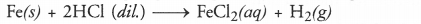Question 4.
What is a balanced chemical equation ? Why should chemical equations be balanced ? (CBSE 2013)
Balanced chemical equation. A chemical equation is said to be balanced if :

1. the atoms of different elements on both sides of the equation are equal.
2. the equation is molecular i.e.. the gases if involved in the equation must be in the molecular form (eg., H2, O2, N2, Cl2 etc.)

Necessity to balance chemical equations. The chemical equations have to be balanced to fulfill the requirement of law of conservation of mass. According to the law,
In a chemical reaction, the mass of reactants must be the same as the mass of products and this is possible only if the atoms of different elements of the reactants and products are equal.

Question 5.
Transfer the following into chemical equations and balance them :
(a) Hydrogen gas combines with nitrogen to form ammonia.
(b) Hydrogen sulphide gas burns in air to give water and sulphur dioxide.
(c) Barium chloride reacts with aluminium sulphate to give aluminium chloride and precipitate of barium sulphate.
(d) Potassium metal reacts with water to give potassium hydroxide and hydrogen gas.
(a) The symbol equation for the reaction is :
H2 + N2 ———> NH3
The balancing of equation is done in the following steps :
Step I: Let us count the number of atoms of all the elements of the reactants and the products on both sides of the equation.

 Elements No. of atoms of reactants      (L.H.S.) No. of atoms of products (R.H.S.) H 2 3 N 2 1

A simple look at the equation reveals that neither the number of H nor of N atoms are equal on both sides of the equation.
Step I: In order to equate the number of H atoms on both sides, put coefficient 3 before H2 on the reactant side and coefficient 2 before NH3 on the product side.
3H2 + N2 ———–> 2NH3
Step III: On counting, the number of N atoms on both sides of the equation are also the same (2). This means that the equation is balanced.
(b) The symbol equation for the reaction is :
H2S + O2 ———–> H2O + SO2
The balancing of equation is done in the following steps :
Step I: Let us count the number of atoms of all the elements on both sides on the equation.

 Elements No. of atoms of reactants (L.H.S.) No. of atoms of products (R.H.S.) H 2 2 S 1 1 O 2 3

A simple look at the equation reveals that the number of H and S atoms are equal on both sides. At the same time, the number of O atoms are not equal.
Step II: In order to equate the number of O atoms, put coefficient 3 before O2 on the reactant side and coefficient 2 before SO2 on the product side.
H2S + 3O2 ———–> H2O + 2SO2
Step III: O atoms are still not balanced. To achieve this, put coefficient 2 before H2O on the product side.
H2S + 3O2 ————> 2H2O + 2SO2
Step IV: To balance S atoms, put coefficient 2 before H2S on the reactant side.
2H2S + 3O2 ————> 2H2O + 2SO2
On inspection, the number of atoms of all the elements in both sides of the equation are equal. Therefore, the equation is balanced.
(c) The symbol equation for the reaction is :
BaCl2 + Al2(SO4)3 ———-> A1Cl3 + BaSO4
The balancing of equation is done in the following steps :
Step I: Let us count the number of atoms of all the elements on both sides of the equation.

 Elements No. of atoms of reactants (L.H.S.) No. of atoms of products (R.H.S.) Ba 1 1 A1 2 1 Cl 2 3 S 3 1 O 12 4

A simple look at the equation reveals that only Ba atoms are equal on both sides. The rest of the atoms are to be balanced. It is done as follows :
Step II: In order to equate the number of Al atoms, put coefficient 2 before AlCl3 on the product side.
BaCl2 + Al2(SO4)3 ———-> 2AICl3 + BaSO4
Step III: In order to balance Cl atoms, put coefficient 3 before BaCl2 on the reactant side.
3BaCl2 + Al2(SO4)3 ———–> 2AlCl3 + BaSO4
Step IV: To balance Ba atoms, put coefficient 3 before BaSO4 on the product side.
3BaCl2 + Al2(SIO4)3 ———-> 2AlCl3 + 3BaSO4
Step V: On inspection, the number of S and O atoms on both sides of the equation are also found to be equal. Thus, the equation is in balanced form.
(d) The symbol equation for the reaction is :
K + H2O ———–> KOH + H2
The balancing of the equation is done in the following steps :
Step I. Let us count the number of atoms of all the elements on both sides.

 Elements No. of atoms of reactants (L.H.S.) No. of atoms of products (R.H.S.) K 1 1 H 2 3 O 1 1

A simple look at the equation reveals that the number of K and O atoms on both sides of the equation are equal. At the same time, the number of H atoms are not equal.
Step II: To balance the number of H atoms, put coefficient 2 before KOH on the product side and 2 before H2O on the reactant side.
K + 2H2O ———> 2KOH + H2
Step III: To balance the number of K atoms in the above equation, put coefficient 2 before K atom on the reactant side.
2K + 2H2O ———> 2KOH + H2
Step IV: On inspection, the number of atoms of all the elements are found to be equal on both sides of the equation. It is balanced.

Question 6.
Balance the following chemical equations :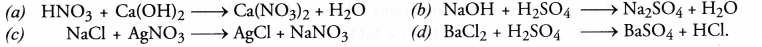(a) The symbol equation as given for the reaction is :
HNO3 + Ca(OH)2 ——–> Ca(NO3)2 + H2O
The balancing of the equation is done in the following steps :
Step I: Let us count the number of atoms of all the elements on both sides of the equation.

 Elements No. of atoms of reactants (L.H.S.) No. of atoms of products (Products) H 3 2 O 5 7 N 1 2 Ca 1 1

A simple look at the equation reveals that the number of Ca atoms are equal on both sides.
Step II: In order to equate the number of N atoms, put coefficient 2 before HNO3 on the reactant side.
2HNO3 + Ca(OH)2 ———-> Ca(NO3)2 + H2O
Step III: In order to equate the number of H atoms, put coefficient 2 before H2O on the product side.
2HNO3 + Ca(OH)2 ———-> Ca(NO3)2 + 2H2O
Step IV: On inspection the number of O atoms on both sides of the equation is the same i.e., 8. Therefore, the equation is balanced.
(b) The symbol equation as given for the reaction is :
NaOH + H2SO4 ———-> Na2SO4 + H2O
Step I: Let us count the number of atoms of all the elements on both sides of the equation.

 Elements No. of atoms of reactants (L.H.S.) No. of atoms of products (R.H.S.) H 3 2 O 5 5 Na 1 2 S 1 1

A simple look at the equation reveals that the number of O and S atoms are equal on both sides.
Step II: In order to equate the number of Na atoms, put coefficient 2 before NaOH on the reactant side.
2NaOH + H2SO4 ———-> Na2SO4 + H2O
Step III: In order to equate the number of H atoms, put coefficient 2 before H2O on the product side.
2NaOH + H2SO4 ———> Na2SO4 + 2H2O
Step IV: On inspection, the number of O atoms on both sides of the equation is the same i.e., 6. Therefore, the equation is balanced.
(c) The symbol equation as given for the reaction is already balanced.
NaCl + AgNO3 ———-> AgCl + NaNO3
On inspection, the number of atoms of all the elements are found to be equal on both sides of the equation. It is in the balanced form.
(d) The symbol equation as given for the reaction is :
BaCl2 + H2SO4 ———-> BaSO4 + HCl
Step I: Let us count the number of atoms of all the elements on both sides of the equation.

 Elements No. of atoms of reactants (L.H.S.) No. of atoms of products (R.H.S.) Ba 1 1 H 2 1 O 4 4 S 1 1 Cl 2 1

A simple look at the equation reveals that the number of Ba, S and O atoms are equal on both sides.
Step II: In order to equate the number of Cl atoms, put coefficient 2 before HCl on the product side.
BaCl2 + H2SO4 ———> BaSO4 + 2HCl
Step III: On inspection, the number of H atoms on both sides of the equation is the same i. e., 2. Therefore, the equation is balanced.

Question 7.
Write the balanced equations for the following reactions :
(a) Calcium hydroxide + Carbon dioxide ———-> Calcium carbonate + Water
(c) Barium chloride + Sodium sulphate ———-> Barium sulphate + Sodium chloride
(d) Zinc + Silver nitrate ———–> Zinc nitrate + Silver.
All these equations are in word form whereas the balanced equations are written in symbol form.
(a) Ca(OH)2 + CO2 ———-> CaCO3 + H2O
(b) Pb + CuCl2 ———-> PbCl2 + Cu
(c) BaCl2 + Na2SO4 ———> BaSO4 + 2NaCl
(d) Zn + 2AgNO3 ———–> Zn(NO3)2 + 2Ag

Question 8.
Write the balanced chemical equations for the following reactions and identify the type of reaction :
(a) Potassium brormide (aq) + Barium iodide (aq) ————> Potassium iodide(aq) + Barium bromide (aq)
(b) Zinc carbonate (aq) ————> Zinc oxide (aq) + Carbon dioxide (aq)
(c) Hydrogen (aq) + Chlorine (aq) ———> Hydrogen chloride (aq)
(d) Magnesium (aq) + Hydrochloric acid (aq) ———–> Magnesium chloride (aq) + Hydrogen(aq).
(a)          2KBr (aq) + BaI(aq) ——–> 2Kl (aq) + BaBr2 (aq)
The reaction is known as double displacement reaction.
(b)          ZnCO3(s) ———-> ZnO(s) + CO2(g)
The reaction is known as decomposition reaction. ‘
(c)          H2(g) + Cl2(g) ———–> 2HCl (g)
The reaction is known as combination reaction.
(d)          Mg (aq) + 2HCl (aq) ———–> MgCl(aq) + H2(g)
The reaction is known as displacement reaction.

Question 9.
What do you mean by exothermic and endothermic reactions ? Give examples.
A chemical reaction is said to be exothermic in which a certain amount of heat energy is released. The container in which reaction is carried gets heated up. For example,
N(g) + 3H2(g) ———> 2NH3 (g) + 92 kj
CH4(g) + 2O2(g) ———> CO(g) + 2H2O (l) + 890 kj
NaOH (aq) + HCl (aq) ———> NaCl (aq)+ H2O (aq) + 57.5 kj
A chemical reaction is said to be endothermic in which a certain amount of heat energy is absorbed. The container in which the reaction is carried becomes cold. For example,
N2(g) + O(g) ———-> 2NO (g) – 180 kj
C(s) + H2O (aq) ———-> CO (g) + H2(g) – 130 kj

Question 10.
Why is respiration considered an exothermic reaction ? (CBSE 2011)
Respiration is the most important biochemical reaction which releases energy in the cells. When we breathe in air, oxygen enters our lungs. It binds itself to haemoglobin present in red cells and is carried to millions of cells present on the body. Respiration occurs in these cells and is accompanied by the combustion of glucose producing carbon dioxide water and heat energy.Question 11.
Why are decomposition reactions called opposite of combination reactions ? Write equations for these reactions.
Combination reaction may be defined as the reaction in which two or more substances combine under suitable conditions to form a new substance. For example,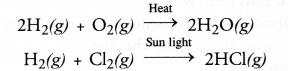A decomposition reaction may be defined as the reaction in which a single substance decomposes or splits into two or more substances under suitable conditions.
For example,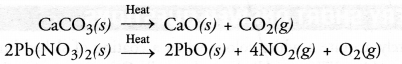It may be concluded that a certain substance is formed or synthesised in combination reaction and it breaks or splits in decomposition reaction. Therefore, the two reactions oppose each other.

Question 12.
Write equations for each decomposition reaction, where energy is supplied in the form of heat, light and electricity.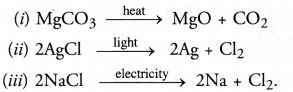Question 13.
What is the difference between displacement and double displacement reactions ? Write equations for these reactions. (CBSE 2011)
In a displacement reaction, one element takes the place of another in a compound dissolved in a solution. For example,
Fe(s) + CuSO4 (aq) ———> FeSO4 (aq) + Cu(s)
In a double displacement reaction, one component each of both the reacting molecules get exchanged to form the products. For example,
HCl (aq) + NaOH(aq) ———> NaCl (aq) + H2O (aq)

Question 14.
In the refining of silver, the recovery of silver from silver nitrate solution involved displacement by copper metal. Write chemical equation involved.
The chemical equation for the displacement reaction is :
Cu(s) + 2AgNO(aq) ———-> Cu(NO3)2 (aq) + 2Ag (s).

Question 15.
What do you mean by precipitation reaction ? Explain giving examples.
In a precipitation reaction, one of the products formed during the reaction does not dissolve in solution and gets settled at the surface of the container (beaker or tube). It is known as a precipitate. For example,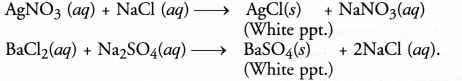Question 16.
Explain the following in terms of gain or loss of oxygen with two examples of each,
(a) oxidation
(b) reduction.
(a) Oxidation involves the gain of oxygen by a substance in a chemical reaction. For example,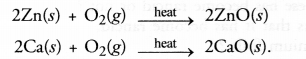(b) Reduction involves the loss of oxygen from a substance in a chemical reaction. For example,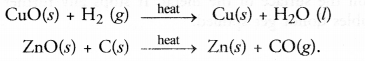Question 17.
A shining brown coloured element ‘X’ on heating in’ air becomes black in colour. Name the element ‘X’ and the black coloured compound formed. (CBSE 2013)
The element ‘X’ is copper and the black coloured compound is copper (II) oxide also known as cupric oxide.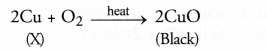Question 18.
Why do you apply paint on iron articles ?
Paint forms a protective coating on the surface of iron. Both oxygen and moisture (present in air) cannot have a direct contact with the surface of the iron metal. Therefore, the surface gets protected against rusting.

Question 19.
Oil and fat containing food items are flushed with nitrogen. Why ?
Oil and fat containing food items or etables get rancid due to oxidation by air or oxygen. In case the container or bag is flushed with nitrogen, then oxidation or rancidity will be checked.

Question 20.
Explain the following terms wih one example of each.
(a) Corrosion
(b) Rusting.
Corrosion may be defined as the chemical process of slow eating up of the surfaces of certain metals when kept in open for a long time.
Quite often, when we open the bonet of a car after a long time, we find a deposit around the terminals of the battery. This is because of corrosion of the terminals. Black coating on the surface of silver and green layer on the surface of copper are the examples of corrosion. In case of iron, corrosion is called rusting. Rust is a chemical substance which is soft and also porous. It is brown in colour and is formed by the chemical action of moist air (containing COand H2O) on iron. It is basically an oxidation reaction and formula of rust is Fe2O3.xH2O. It is very slow in nature and once started keeps on.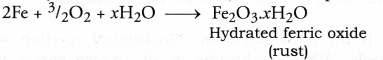Both corrosion and rusting are very harmful and cause damage to the buildings, railway tracks, automobiles and other objects/materials where metals are used. We quite often hear that an old building has collapsed of its own causing loss of both lives and property. This is on account of the rusting of iron which is used in making the structures particularly the roof. Corrosion also causes constant damage to the statues made up of marble which is chemically calcium carbonate (CaCO3). Both sulphuric acid and nitric acid present in the rain water dissolve calcium carbonate to form calcium sulphate and calcium nitrate respectively.
CaCO3 + H2SO———— > CaSO4 + CO2 + H2O

CaCO3 + 2HNO3 ———— > Ca(NO3)2 + CO2 + H2O

In addition to this, traces of hydrogen sulphide gas (H2S) present in atmosphere form black stains on these statues due to calcium sulphide which is black in colour.
CaCO3 + H2S  ———–> CaS + H2O + CO2
Corrosion has caused huge damage to our historical monuments including ‘Taj Mahal’ which is regarded as the eighth wonder. Marble is chemically CaCO3. Polluted air contains both H2SO4 and HNO3 along with traces of H2S gas. They react chemically with CaCO3 as shown above. As a result, this historical monument which is the pride of India is being constancy corroded. Every effort must be made to protect ‘Taj Mahal’ and other similar monuments which are our treasure. Corrosion (or rusting) occurs in metals only. We shall study it detail and the measures to check corrosion in chapter 3 on Metals and Non-Metals.

NCERT Solutions for Class 10 Science Chapter 1 Chemical Reactions and Equations

Hope given NCERT Solutions for Class 10 Science Chapter 1 are helpful to complete your science homework.

If you have any doubts, please comment below. Learn Insta try to provide online science tutoring for you.